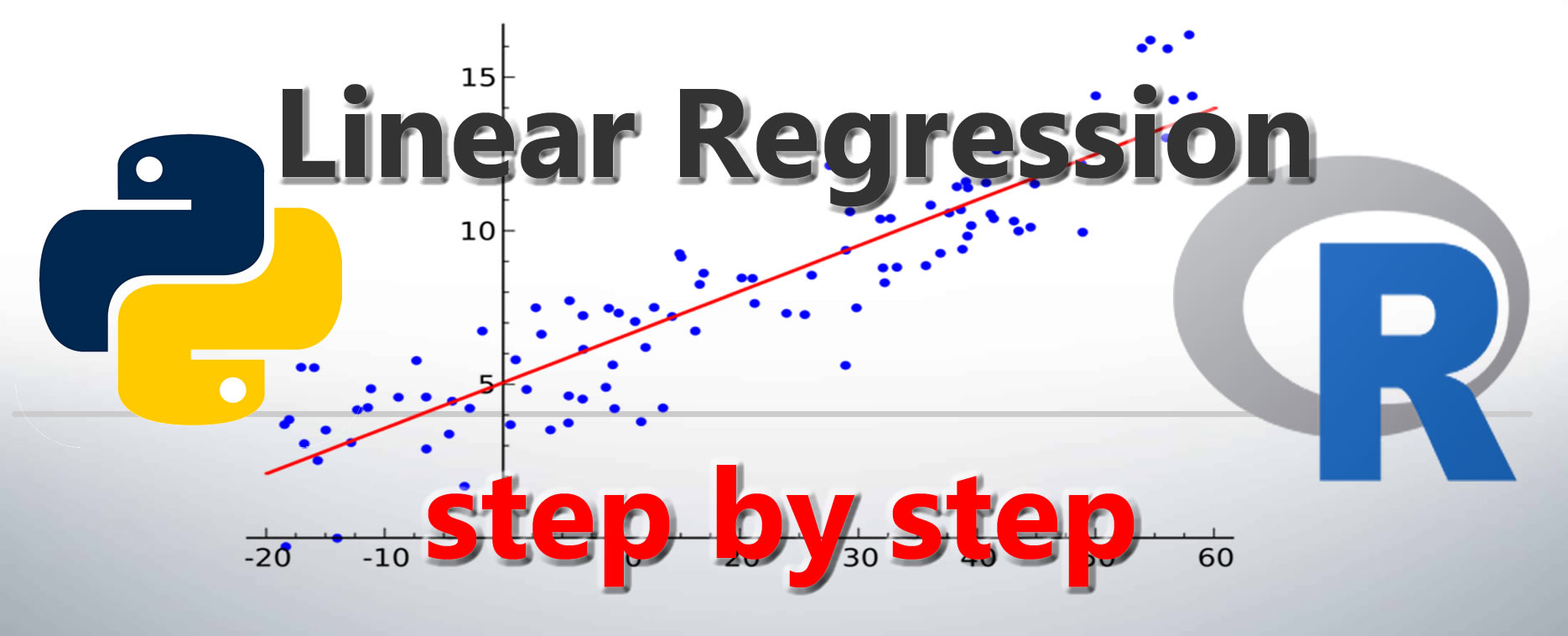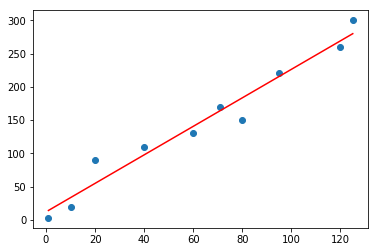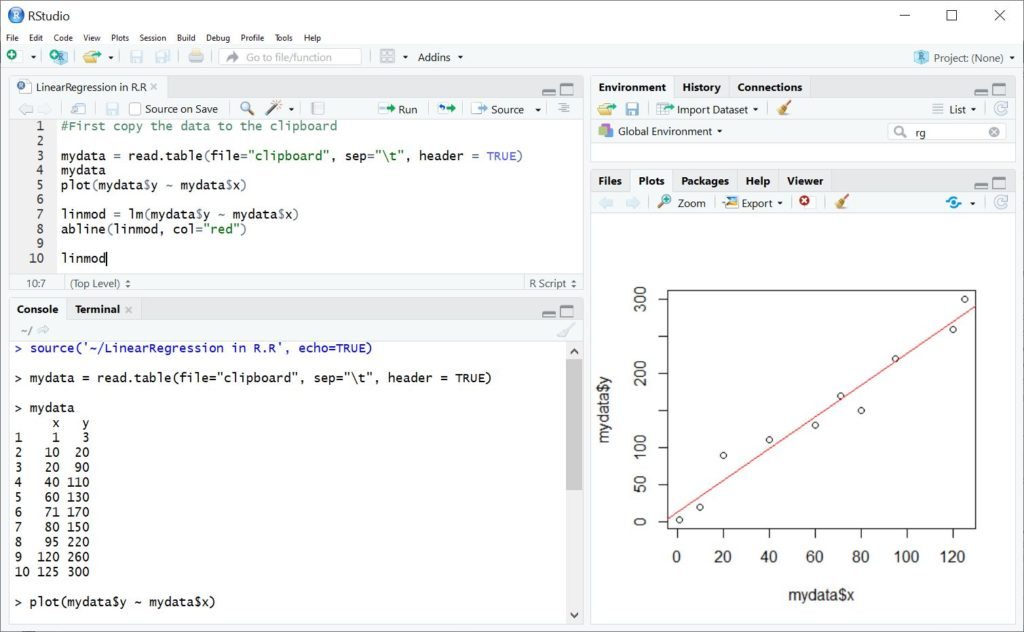# How to Perform Linear Regression in Python and R( Similar Results)

In this short lesson, I would teach you how to perform linear regression in Python and R. It’s quite easy. But the interesting thing is that we get similar results.

##### Linear Regression in Python

Now the data is given in an excel spreadsheet. This is shown below:

 x y 1 3 10 20 20 90 40 110 60 130 71 170 80 150 95 220 120 260 125 300

You can watch the video to see how to easily transfer this data to Jupyter Notebook.

The Python code is shown below. I have also included comments in the code to make it easily readable.

```#Import the necessary modules
import numpy as np
import matplotlib.pyplot as plt
from sklearn.linear_model import LinearRegression

#Create a numpy array using the given dataset
x = np.array([1,10,20,40,60,71,80,95,120,125])
y = np.array([3,20,90,110,130,170,150,220,260,300])

#Create a LinearRegression variable
linmod = LinearRegression()
x = x.reshape(-1, 1)
linmod.fit(x,y)
y_pred = linmod.predict(x)

#First do a scatterplot, then fit a regression line
plt.scatter(x,y)
plt.plot(x, y_pred, color='red')
plt.show()
``````print(linmod.intercept_)
12.054491647432457

print(linmod.coef_)
[2.14221075]
```

Just in case you miss out anything, the Jupyter  Notebook window is shown below.

##### Linear Regression in R

Let’s now examine how to perform Linear Regression in R. I’m sure you know you need to have RStudio installed.

The whole code is given below.

```#First copy the data to the clipboard

mydata
plot(mydata\$y ~ mydata\$x)

linmod = lm(mydata\$y ~ mydata\$x)
abline(linmod, col="red")

linmod
```

The graph generated by R is shown below

The RStudio window is shown below:I would recommend you watch the video lesson below:

You can also find series of R Tutorials below:

Tutorial 1. R Setup and Your Firs Commands – https://youtu.be/ri1tmqEGn-E
Tutorial 2. R Script and Matrices – https://youtu.be/Vonuc47oEWQ
Tutorial 3. Indexing Matrices and Dataset – https://youtu.be/p-JrNg7InUE
Tutorial 4. Importing Data into R – https://youtu.be/p-JrNg7InUE
Tutorial 5. Plotting in R (2D) – https://youtu.be/pz1mH5q9Jnw
Tutorial 6. Creating Interactive 3D Plots – https://youtu.be/9VmXQSfD9os
How to Perform Linear Regression in R – https://youtu.be/MNHaXSZVceo
How to Perform Linear Regression in Python – https://youtu.be/iaom_n2ER-Q

Subscribe Kindson The Genius Youtube: https://bit.ly/2PpJd8Q
Join Machine Learning & Data Science in Python and R – https://www.facebook.com/groups/704770263315075/
Join my group ICS on Facebook: https://bit.ly/2UULiQj
Follow me on Instagram – https://www.instagram.com/kindsonm/

R Tutorials for Data Science and Machine Learning#### kindsonthegenius

Kindson Munonye is currently completing his doctoral program in Software Engineering in Budapest University of Technology and Economics

View all posts by kindsonthegenius →

## One thought on “How to Perform Linear Regression in Python and R( Similar Results)”

1.Moving company in Virginia says:

This post gives clear idea designed for the new visitors of blogging, that truly how to do running a blog.